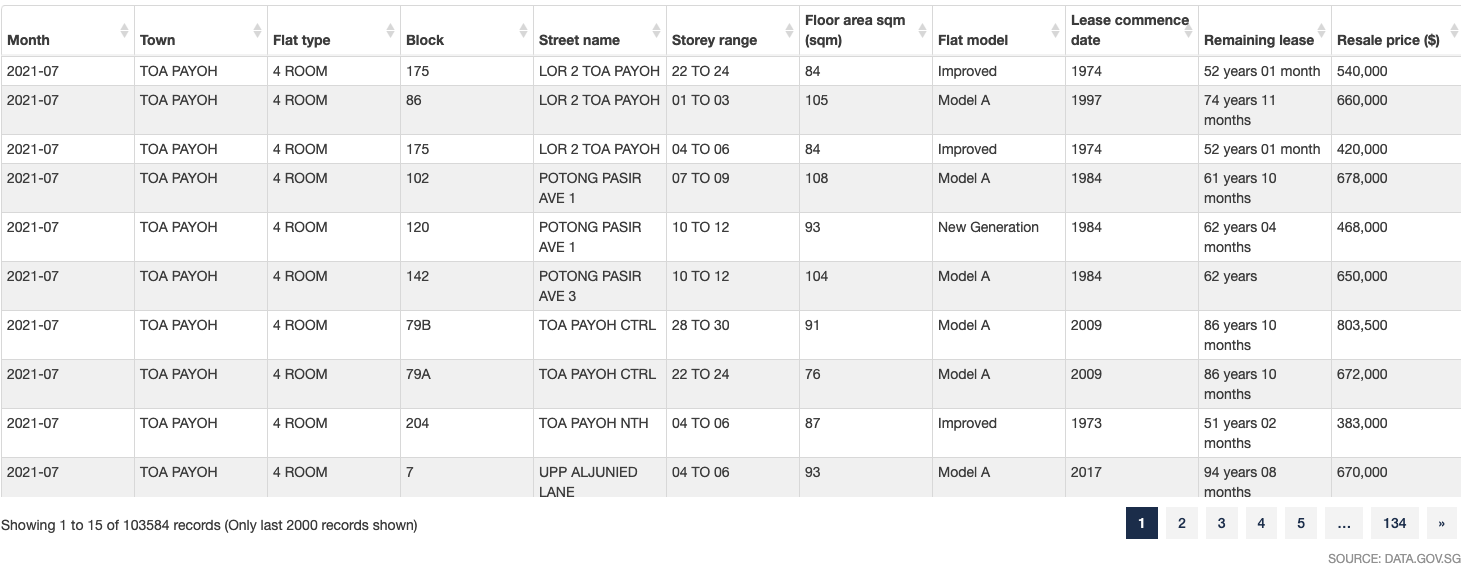# Machine Learning in a nutshell

A simple explanation of the use of machine learning, is the prediction of result based on past data. In order to do that, you’ll need a lot of data, because the more data you have, the more information you can make use to predict.

Usually you’ll have a table of data, with many fields, of which one of the fields is the data you’ll want to predict. As in the screenshot of the table of public housing resale price in Singapore, the `Resale price` is what we want to predict, which we refer to as the `target`.However, not all of the other fields are relevant to be used to predict the answer we wanted, so we must still exercise some human intelligence (and sometimes common sense) to only choose the relevant ones. For example, the `Block` field is most likely irrelevant. For the fields we chose, we refer to them as `features`.

Now, to get into the business of prediction, as what you might have heard someone say before, is that we need to define a model to train our data. What does that actually mean? Well, a model is like a formula, something mathematical, where you can provide your input values, and the formula will generate a result for you. But in the case of machine learning, since every dataset is different, you don’t have to write the formula yourself. Instead, you use a formula to create a formula for your dataset to predict the result. We call this `formula to create formula` a `model`. Generally, a model will run the data with all sorts of permutations and combinations, and get you the formula with the best result, in the most efficient way it can. In order to do that, you need to provide the model what we call training data, which are the feature data and its respective target data, as mentioned above.

There are quite a number of models, and each has its own pros and cons, suitable for different types of data. But they are plug-and-play, you just need to apply it. Like how you can drive a car without the need to understand how the car works, but you still need to understand basically what type of data is it good for. For example, if you are going to drive off-roads, you’ll need a SUV. But if you are just driving in the city with heavy traffic, a normal sedan might be more economical.

``````Input -> Process -> Output
``````

As in the classic input -> process -> output, we need to supply the input and output, so the model can provide the process. We call this `fitting` Once we got the process, we provide the input to the process, to `predict` the output.

Lastly, models aren’t perfect. Sometimes the formula is too long and detailed, it can mistook outliers as normal. Sometimes, the formula is too short, it is not extensive enough to be accurate. We can evaluate how good the model is by calculating how much/big the errors are, and run the model a few times with different settings, to find the best model with the least error.

The above scenario is an example of supervised learning, a subset of the machine learning spectrum. It is meant to describe how machine learning works in basic layman terms (as much as I can). There are much more to it, like unsupervised learning, difference between classification and regression, the different models available, which i’ll leave for next time.

Tags:

Categories:

Updated: